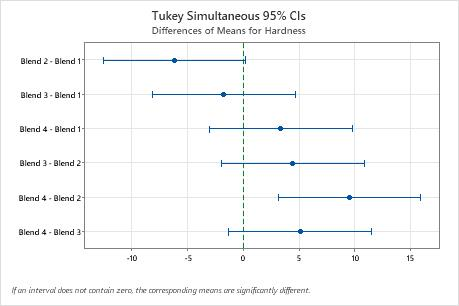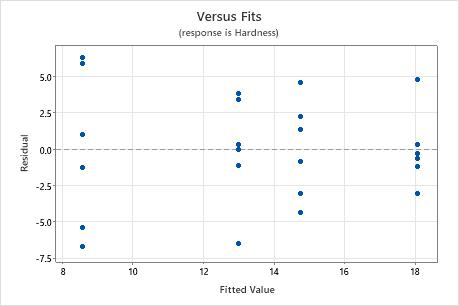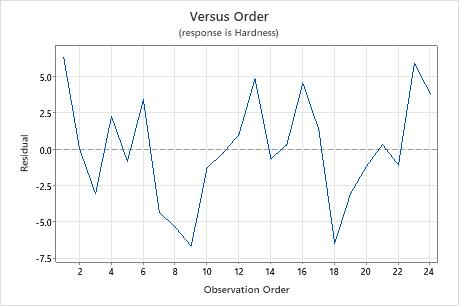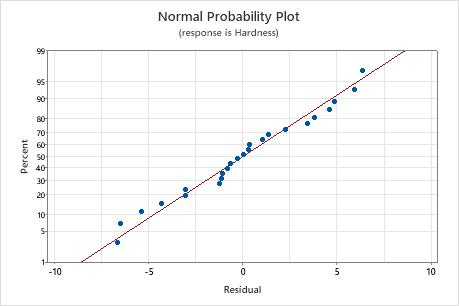# Interpret the key results for One-Way ANOVA

Complete the following steps to interpret One-Way ANOVA. Key output includes the p-value, the graphs of groups, the group comparisons, R2, and the residual plots.

## Step 1: Determine whether the differences between group means are statistically significant

To determine whether any of the differences between the means are statistically significant, compare the p-value to your significance level to assess the null hypothesis. The null hypothesis states that the population means are all equal. Usually, a significance level (denoted as α or alpha) of 0.05 works well. A significance level of 0.05 indicates a 5% risk of concluding that a difference exists when there is no actual difference.
P-value ≤ α: The differences between some of the means are statistically significant
If the p-value is less than or equal to the significance level, you reject the null hypothesis and conclude that not all population means are equal. Use your specialized knowledge to determine whether the differences are practically significant. For more information, go to Statistical and practical significance.
P-value > α: The differences between the means are not statistically significant
If the p-value is greater than the significance level, you do not have enough evidence to reject the null hypothesis that the population means are all equal. Verify that your test has enough power to detect a difference that is practically significant. For more information, go to Increase the power of a hypothesis test.

## Step 2: Examine the group means

Use the interval plot to display the mean and confidence interval for each group.

The interval plots show the following:
• Each dot represents a sample mean.
• Each interval is a 95% confidence interval for the mean of a group. You can be 95% confident that a group mean is within the group's confidence interval.
###### Important

Interpret these intervals carefully because making multiple comparisons increases the type 1 error rate. That is, when you increase the number of comparisons, you also increase the probability that at least one comparison will incorrectly conclude that one of the observed differences is significantly different.

To assess the differences that appear on this plot, use the grouping information table and other comparisons output (shown in step 3).In the interval plot, Blend 2 has the lowest mean and Blend 4 has the highest. You cannot determine from this graph whether any differences are statistically significant. To determine statistical significance, assess the confidence intervals for the differences of means.

## Step 3: Compare the group means

If your one-way ANOVA p-value is less than your significance level, you know that some of the group means are different, but not which pairs of groups. Use the grouping information table and tests for differences of means to determine whether the mean difference between specific pairs of groups are statistically significant and to estimate by how much they are different.

For more information on comparison methods, go to Using multiple comparisons to assess the practical and statistical significance.

Grouping Information table

Use the grouping information table to quickly determine whether the mean difference between any pair of groups is statistically significant.

Groups that do not share a letter are significantly different.

Tests for differences of means

Use the confidence intervals to determine likely ranges for the differences and to determine whether the differences are practically significant. The table displays a set of confidence intervals for the difference between pairs of means. The interval plot for differences of means displays the same information.

Confidence intervals that do not contain zero indicate a mean difference that is statistically significant.

Depending on the comparison method you chose, the table compares different pairs of groups and displays one of the following types of confidence intervals.
• Individual confidence level

The percentage of times that a single confidence interval includes the true difference between one pair of group means, if you repeat the study multiple times.

• Simultaneous confidence level

The percentage of times that a set of confidence intervals includes the true differences for all group comparisons, if you repeat the study multiple times.

Controlling the simultaneous confidence level is particularly important when you perform multiple comparisons. If you do not control the simultaneous confidence level, the chance that at least one confidence interval does not contain the true difference increases with the number of comparisons.

For more information, go to Understanding individual and simultaneous confidence levels in multiple comparisons.

For more information about how to interpret the results for Hsu's MCB, go to What is Hsu's multiple comparisons with the best (MCB)?Key Results: Simultaneous 95% CIs, Individual confidence level In the Tukey results, the confidence intervals indicate the following: The confidence interval for the difference between the means of Blend 2 and 4 is 3.11 to 15.89. This range does not include zero, which indicates that the difference is statistically significant. The confidence intervals for the remaining pairs of means all include zero, which indicates that the differences are not statistically significant. The 95% simultaneous confidence level indicates that you can be 95% confident that all the confidence intervals contain the true differences. The table indicates that the individual confidence level is 98.89%. This result indicates that you can be 98.89% confident that each individual interval contains the true difference between a specific pair of group means. The individual confidence levels for each comparison produce the 95% simultaneous confidence level for all six comparisons.

## Step 4: Determine how well the model fits your data

To determine how well the model fits your data, examine the goodness-of-fit statistics in the Model Summary table.

S
Use S to assess how well the model describes the response.

S is measured in the units of the response variable and represents how far the data values fall from the fitted values. The lower the value of S, the better the model describes the response. However, a low S value by itself does not indicate that the model meets the model assumptions. You should check the residual plots to verify the assumptions.

R-sq

R2 is the percentage of variation in the response that is explained by the model. The higher the R2 value, the better the model fits your data. R2 is always between 0% and 100%.

A high R2 value does not indicate that the model meets the model assumptions. You should check the residual plots to verify the assumptions.

R-sq (pred)

Use predicted R2 to determine how well your model predicts the response for new observations. Models that have larger predicted R2 values have better predictive ability.

A predicted R2 that is substantially less than R2 may indicate that the model is over-fit. An over-fit model occurs when you add terms for effects that are not important in the population. The model becomes tailored to the sample data and, therefore, may not be useful for making predictions about the population.

Predicted R2 can also be more useful than adjusted R2 for comparing models because it is calculated with observations that are not included in the model calculation.

## Step 5: Determine whether your model meets the assumptions of the analysis

Use the residual plots to help you determine whether the model is adequate and meets the assumptions of the analysis. If the assumptions are not met, the model may not fit the data well and you should use caution when you interpret the results.

### Residuals versus fits plot

Use the residuals versus fits plot to verify the assumption that the residuals are randomly distributed and have constant variance. Ideally, the points should fall randomly on both sides of 0, with no recognizable patterns in the points.

The patterns in the following table may indicate that the model does not meet the model assumptions.
Pattern What the pattern may indicate
Fanning or uneven spreading of residuals across fitted values Nonconstant variance
A point that is far away from zero An outlier
In this residual versus fits plot, the points appear randomly scattered on the plot. None of the groups appear to have substantially different variability and no outliers are apparent.### Residuals versus order plot

Use the residuals versus order plot to verify the assumption that the residuals are independent from one another. Independent residuals show no trends or patterns when displayed in time order. Patterns in the points may indicate that residuals near each other may be correlated, and thus, not independent. Ideally, the residuals on the plot should fall randomly around the center line:If you see a pattern, investigate the cause. The following types of patterns may indicate that the residuals are dependent.
In this residual versus order plot, the residuals fall randomly around the centerline.### Normal probability plot of the residuals

Use the normal probability plot of the residuals to verify the assumption that the residuals are normally distributed. The normal probability plot of the residuals should approximately follow a straight line.

The patterns in the following table may indicate that the model does not meet the model assumptions.
Pattern What the pattern may indicate
Not a straight line Nonnormality
A point that is far away from the line An outlier
Changing slope An unidentified variable
###### Note

If your one-way ANOVA design meets the guidelines for sample size, the results are not substantially affected by departures from normality.

In this normal probability plot, the residuals appear to generally follow a straight line. From the residuals versus fits plot, you can see that there are six observations in each of the four groups. Because this design does not meet the sample size guidelines, it is important to satisfy the normality assumption so that the test results are reliable.By using this site you agree to the use of cookies for analytics and personalized content.  Read our policy